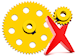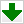"Stellated Dodecahedron" by EDV - 09/11/2015
144.443 bytes - 630 parts
Description
This is a concave convex solid whose facets are defined by the extension of the edges of a regular dodecahedron.

It contains 12 x 5 pointed star faces enhanced in the model using different colors for each.

The regular dodecahedron central core is enhanced by coloring white all the strips positioned along the central core.

This Meccano model is a good close approximation to the shape of a real regular stellated dodecahedron since it has a ratio of lenght of its short and long edges of 5 to 8 while the real one has a ratio of 5 to 8.0902

Its facets are identical to the ones used on the model drawn for the stellated icosahedron since the dodecahedron and icosahedron are related where one has 12 pentagonal faces and 20 vertices and the other has 20 triangular faces and 12 vertices. The total of edges on both count 60 onits.

On the real regular stellated dodecahedron both the dihedral angle between facets or the one between pyramids measure 116.565º.

On the Meccano model the dihedral angle between facets is 116.788º and the one between pyramids is 117.281º

When building the real thing the obtuse angle brackets used will deform to the correct angle as the bolts are fastenedDownload model (.mdl)